Disclaimer: This is an example of a student written essay.

Any scientific information contained within this essay should not be treated as fact, this content is to be used for educational purposes only and may contain factual inaccuracies or be out of date.

# Determining Effects on Temperature on the Solubility of an Acid through Acid-Base Titrations

 ✅ Paper Type: Free Essay ✅ Subject: Chemistry ✅ Wordcount: 1424 words ✅ Published: 3rd Nov 2020

Theory

The goal of the experiment was to be able to determine the effect that temperature has on solubility by using a salt in water and doing acid-base titrations at varying temperatures. The experiment will also be done to examine the relationship of solubility and enthalpy. The definition of the solubility of a substance is known as the number of grams of a salt that will dissolve in 100 grams of water. The titrant used to determine the concentrations of the analyte, Benzoic Acid, was Sodium Hydroxide. This was done by titration trials under different temperatures. The titration can be expressed as an equation: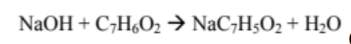The equation shown below is used to determine the enthalpy (H), using the solubility terms (S), the gas constant (R) and the different temperatures (T), from given literature values: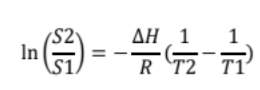For the graphs that will be used to examine the data, an equation will be used to calculate change in enthalpy and plotted: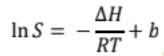Results

Table 1: Displaying volume of titrant used and calculated solubility values at varying temperatures

 Temperature (°C) Trials Volume of Titrant used (mL) Average Volume (mL) Density (g/mL) Solubility in Molarity (M) Solubility in grams (g/100 H2O) 50.0 1 32.7 32.3 0.99803 0.00646 0.1295 2 34.2 3 29.9 45.8 1 29.5 30.2 0.98988 0.00604 0.12203 2 31.5 3 29.5 40.8 1 25.9 27.3 0.99191 0.00546 0.1103 2 31.5 3 24.4 35.8 1 24.0 23.6 0.99376 0.00472 0.09499 2 23.7 3 23.0 30.8 1 18.5 18.2 0.99541 0.00364 0.07314 2 17.3 3 18.7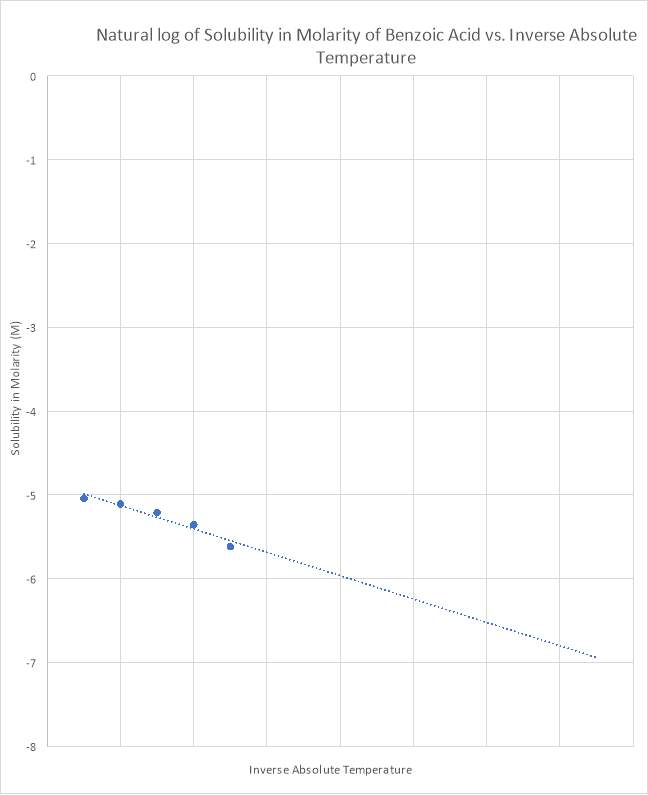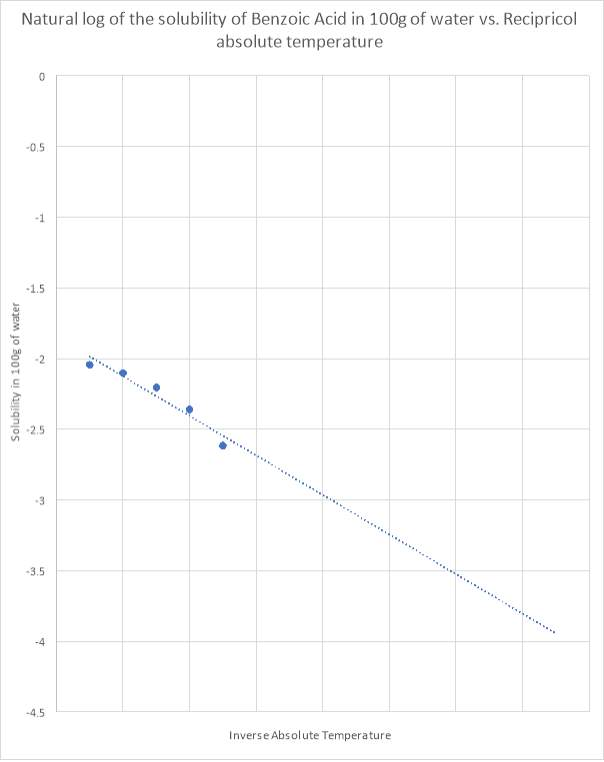Discussion

1) Another method to measure the solubility of mass would be by weighing out salt and recording the initial mass before placing it on a watch glass. Then creating a 100mL distilled water solution and pouring the salt in it. Next, weigh a beaker before and after the solution is poured inside. Then measure the temperature of the solution and take the final mass of the beaker. The mass of the salt can then be determined by subtracting the final mass by the initial mass. From this information the solubility in grams of salt per 100 grams of water can be determined.

Our academic experts are ready and waiting to assist with any writing project you may have. From simple essay plans, through to full dissertations, you can guarantee we have a service perfectly matched to your needs.

2) The experiment consisted of a few experimental and random errors. A main error that could have caused a significant effect to the data could have been the cotton not being able to filter out the solids fully. This could affect the equilibrium of the system, resulting in more titrant being used causing inaccuracies in the data. Other errors could have consisted of the amount of titrant and analyte used, and consistency in reading the burette.

3) A saturated solution is at its final state when no more solute can be dissolved in a certain amount of solvent. In this experiment however, the solute was dissolved prior to the titration resulting in the solution to be at equilibrium. Adding more solute could verify that no more can be dissolved and would show the solution is saturated and at equilibrium.

4)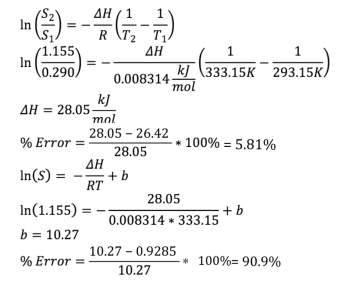Using the graphs, the value for delta H was obtained as 26.42 kJ/mol and b as 0.9285. The literature value for delta H is 28.05 and b is 10.27. The difference in the literature and experimental can be noted. This may be due to errors such as the ones listed above. The percent error is calculated to be 5.81% for the enthalpy and 90.9% for the b value. These values are significant due to the fact that they represent the heat difference and the starting enthalpy.

Appendix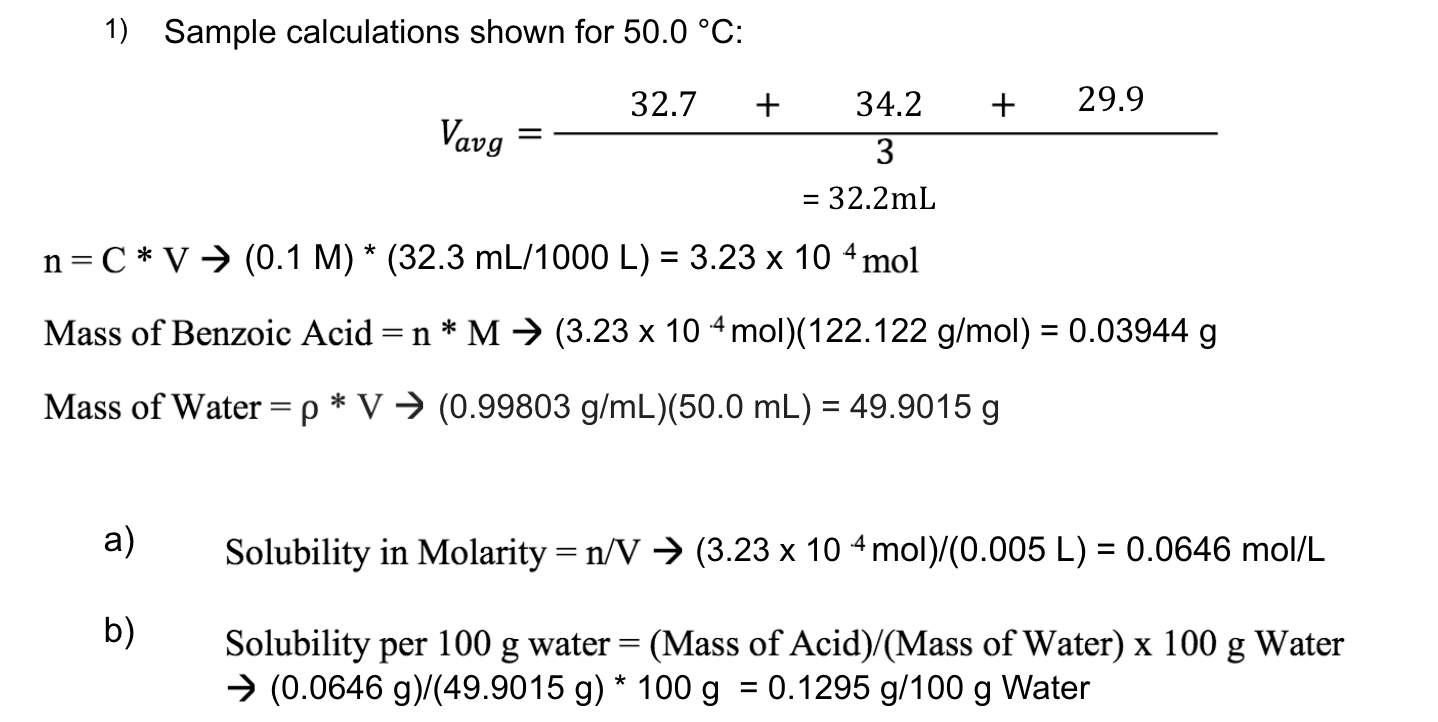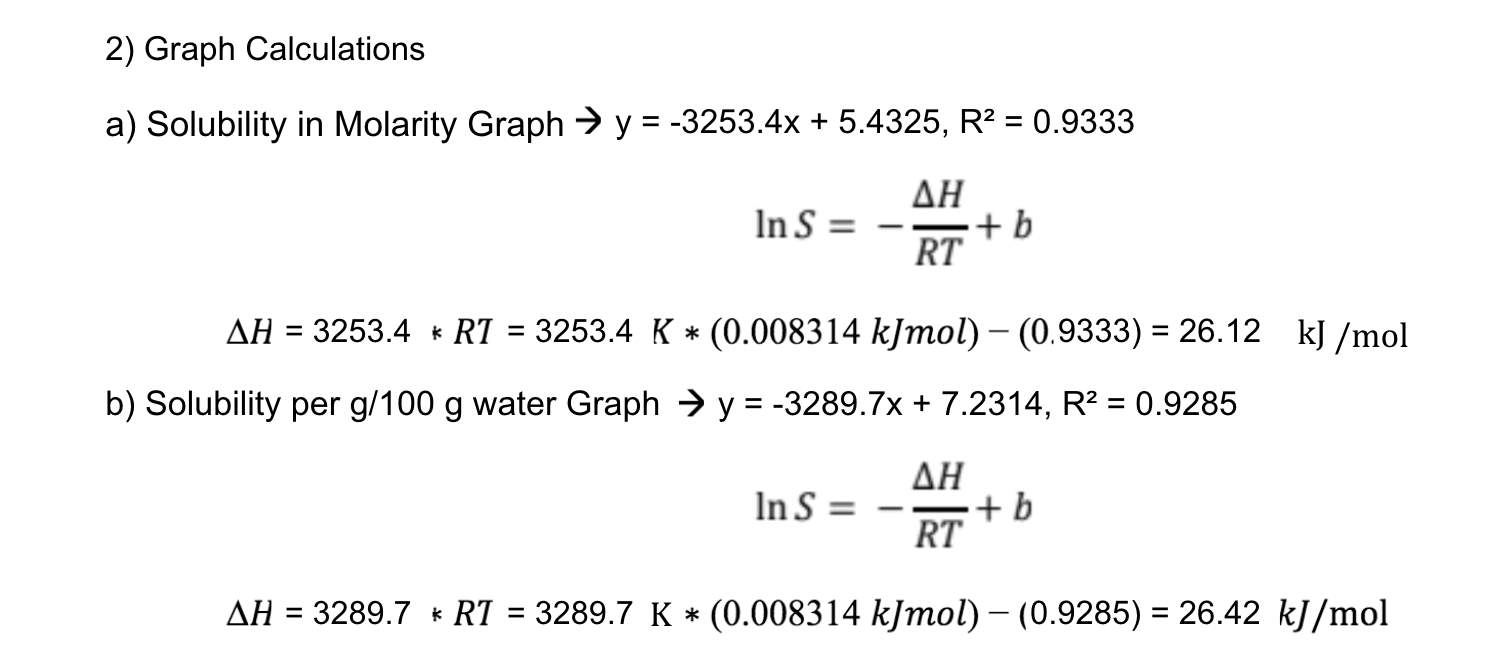Conclusion

Therefore, a conclusion can be made that the solubility of benzoic acid increases with an increase in temperature. This would mean that the benzoic acid is more soluble in water. The solubility of the benzoic acid in water was found to be the following: 0.07905g, 0.07456, 0.06736g, 0.05768g, 0.04466g per 100 grams of water at 50.0°C, 45.8°C, 40.8°C, 35.8°C, and 30.8°C respectively. The solubility of the benzoic acid and sodium hydroxide was observed through titrations at each temperature. The differential heat was calculated to be 26.42 kJ/mol compared to the literature value which was calculated to be 28.05 kJ/mol. This may have occurred because of experimental and human errors throughout the experiment. The percent error was found to be 5.81%which represents a fair accuracy of the lab. Due to errors such as the filtrate not being able to fully filtrate the acid solids, the accuracy of the lab could not be higher, which would result in more accurate values and similar values to the literature values calculated. Overall, the lab could be considered a success as it established its objective of being able to determine the effect of temperature on the solubility of benzoic acid in water.

References

1. Department of Chemistry and Biology Ryerson University. CHE 214 Laboratory Manual for Thermodynamics II. Toronto ON: Ryerson, 2020.
2. Klein, David, Organic Chemistry. Second ed. United States of America: John Wiley and Sons, Inc. 2014.Print.

View all

## DMCA / Removal Request

If you are the original writer of this essay and no longer wish to have your work published on UKEssays.com then please:

Related Services

Related Lectures